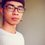# Help me!

$(x+1)( x^{2}+1)=( \sqrt{x+1}+1)(x+2)$Note by Cong Thanh
5 years, 1 month ago

This discussion board is a place to discuss our Daily Challenges and the math and science related to those challenges. Explanations are more than just a solution — they should explain the steps and thinking strategies that you used to obtain the solution. Comments should further the discussion of math and science.

When posting on Brilliant:

• Use the emojis to react to an explanation, whether you're congratulating a job well done , or just really confused .
• Ask specific questions about the challenge or the steps in somebody's explanation. Well-posed questions can add a lot to the discussion, but posting "I don't understand!" doesn't help anyone.
• Try to contribute something new to the discussion, whether it is an extension, generalization or other idea related to the challenge.

MarkdownAppears as
*italics* or _italics_ italics
**bold** or __bold__ bold
- bulleted- list
• bulleted
• list
1. numbered2. list
1. numbered
2. list
Note: you must add a full line of space before and after lists for them to show up correctly
paragraph 1paragraph 2

paragraph 1

paragraph 2

[example link](https://brilliant.org)example link
> This is a quote
This is a quote
    # I indented these lines
# 4 spaces, and now they show
# up as a code block.

print "hello world"
# I indented these lines
# 4 spaces, and now they show
# up as a code block.

print "hello world"
MathAppears as
Remember to wrap math in $$ ... $$ or $ ... $ to ensure proper formatting.
2 \times 3 $2 \times 3$
2^{34} $2^{34}$
a_{i-1} $a_{i-1}$
\frac{2}{3} $\frac{2}{3}$
\sqrt{2} $\sqrt{2}$
\sum_{i=1}^3 $\sum_{i=1}^3$
\sin \theta $\sin \theta$
\boxed{123} $\boxed{123}$

Sort by:

What have you tried? Have you tried squaring the equation? The only real solution is $\dfrac{1+\sqrt5}2$.

- 5 years, 1 month ago

Can you solve it in steps? It also has $(1- \sqrt{5})/2$

- 5 years, 1 month ago

Oh right, I forgot to count the negative sign.

Rearrange the equation: $\dfrac{(x+1)(x^2+1)}{x+2} - 1 = \sqrt{x+1}$. Now square both sides of the equation and simplify it.

You will get the equation $x^6+2 x^5+x^4-3 x^3-7 x^2-8 x-3 = 0$. Factorize it and show that it only has two real roots: $\dfrac{1\pm\sqrt5}2$.

- 5 years, 1 month ago

Thank for your help! My friend solves it by $f(x)=f( \sqrt{x+1})$ $( x+1)( x^{2}+1)=( \sqrt{x+1}+1)( \sqrt{x+1}^{2}+1)$ It also is $f(a)=(a^{2}+1)(a+1)$ <=> $x= \sqrt{x+1} => x^{2}-x-1=0$

- 5 years, 1 month ago

That's a very nice approach! But the thing is, you didn't prove that they are the only solutions. It make sense that if $f(x) = f(\sqrt{x+1})$, then $x = \sqrt{x+1}$, but does that mean that they are the only solution?

- 5 years, 1 month ago

This comes from the one-one nature of $f(x)$.

$f(x)=x^3+x^2+x+1$ Since $f(x)$ is a odd degree polynomial, for proving $f(x)$ to be one-one, it suffices to prove $f(x)$ is monotonically increasing.

$f'(x)=3x^2+2x+1>0\forall x\in\mathfrak R$ ( This comes from the fact that discriminant of $f'(x)$ is $-ve$.)

Hence $f(x_1)=f(x_2)\iff x_1=x_2\forall x_1,x_2\in\mathfrak R$.

Is it right @Pi Han Goh ?

- 5 years, 1 month ago

You don't need to use quadratic discriminant. Other than that, your solution is perfect.

- 5 years, 1 month ago

Are you talking abt alternate method to prove one-one nature of $f(x)$?

- 5 years, 1 month ago

No no. You're missing the point here. the domain for $x$ is $x>-1$ right? Just show that $3x^2 + 2x + 1$ is strictly positive and you're done.

Of course you can use quad discriminant as well....

- 5 years, 1 month ago

Oh that way... Oops :-)

- 5 years, 1 month ago

I don't know but i know you can prove it!

- 5 years, 1 month ago